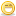CodeGuru Home VC++ / MFC / C++ .NET / C# Visual Basic VB Forums Developer.com

1.Junior MemberJoin Date
Apr 2008
Posts
3

## C++ help needed

I need help finding cos, sin, and tan of an angle. I cannot use the intrinsic function COS,SIN already in C++. Instead we have to use cos (x+y)= cos(x)cos(y)-sin(x)sin(y) and cos^2(x)+sin^2(x)= 1, to find the cos and sin of the angle.(I'm really confused on this)

This is what I got so far:

//Finds sin,cos,tan of angle and quadrant
#include <iostream>
#include <cmath>
using namespace std;

int main ()
{

double angle, sin, cos, tan, quadrant, end;

cout << "Enter angle.\n";
cin >> angle;

while (angle > 360)
{
angle = angle - 360;
}

if (angle <= 90)
cout << "angle is in Quadrant 1\n";
else if ((angle <= 180) && (angle >= 91))
cout << "angle is in Quadrant 2\n";
else if ((angle <= 270) && (angle >= 181))
cout << "angle is in quadrant 3\n";
else if ((angle <= 360) && (angle >= 271))
cout << "angle is in quadrant 4\n";

Any help would be appreciated. Thanks!

So if you know that cos(1) = 0.9998477, then by using cos^2(x)+sin^2(x)=1 you can get to sin^2(1). You need to decide if it is positive or negative, since 1 degree is in the first quadrant, then its sin must be positive, so it will be sin=sqrt(1-0.9998477^2).

From cos(1) and sin(1) you can get cos(1+1), from the first equation. Then you can get sin (2). You can repeat this until you get all the cos and sin for all the angles in 1 degree increment.Reply With Quote

2.Junior MemberJoin Date
Apr 2008
Posts
1

##Re: C++ help needed

When converting a double to an integer type, everything following the decimal is truncated. For finding the quadrant you might consider:
Code:
`int quadrant=1+angle/90;`
(I too am ignoring the possibility of negative angles for this exercise).

Knowing the quadrant, you should probably change the angle by decrements of 90 until it is bounded from above by 90 (the signs can be determined from the quadrant alone).

For the input angles, are you only supposed to admit integers? At any rate, you could cache computed values in an STL vector or an array (a simple, worthwhile exercise over the integers), or you could use a recursive construct (which probably makes more sense for a program like this).

For the recursive route, your function prototypes could look like:
Code:
```double computeCosine(int remainingAngle, double partialCosine);
double computeSine(double Cosine);```
I've changed that angle to type int here.

Also, you can handle the possibility of negative angles very simply (sorry, I can't help myself):
Code:
```if (angle < 0)
{
angle=-angle;
} else
{
}```
Hope this gets you started and good luck.Reply With Quote

3.Junior MemberJoin Date
Apr 2008
Posts
3

## Re: C++ help needed

Thank you.Reply With Quote

####Posting Permissions

• You may not post new threads
• You may not post replies
• You may not post attachments
• You may not edit your posts
•